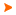Podaci o VU Početna stranicaArhiva nastavnih programaArhiva ak. kalendaraNumerical Methods in Continuum Mechanics
 Abbreviation: NMUMK Load: 30(L) + 15(E) + 0(LE) + 0(CE) + 0(PEE) + 0(FE) + 0(S) + 0(DE) + 0(P) + 0(FLE) + 0()
 Lecturers in charge: prof. dr. sc. Neven Duićprof. dr. sc. Hrvoje Jasakdoc. dr. sc. Milan Vujanović Lecturers: Tessa Uroić mag. ing. mech. ( Exercises ) doc. dr. sc. Milan Vujanović ( Exercises ) Course description: Course objectives: Introduction to the numerical methods used in continuum mechanics, with an emphasis on numerical modelling of heat and mass transfer. The main objective of the course is to provide knowledge necessary for the mathematical modelling of heat and mass transfer and numerical simulations, which then can serve for a better understanding of real processes in modern engineering systems.Enrolment requirements and required entry competences for the course: Thermodynamics I, Fluid Dynamics IStudent responsibilities: Regular attendance at the classes (the lectures and computer exercises). Independent solving and successful submission of the project tasks assigned during the classes.Grading and evaluation of student work over the course of instruction and at a final exam: Ocjenjivanje programskih zadataka (45%), ocjenjivanje uspjeha postignutog na usmenom ispita (55%).Methods of monitoring quality that ensure acquisition of exit competences: Monitoring of the student attendance at the classes, interactive teaching process with active student participation in discussions during lectures and laboratory exercises. Project is used to assess the student"s ability for modelling and solving real practical engieering problems. Evaluation of the student presentation of the project. Evaluation of the results achieved at oral exam. Consultations. Student questionnaires.Upon successful completion of the course, students will be able to (learning outcomes): apply conservation laws; apply Finite Volume Method to the fluid flow analysis; generate a mesh for Finite Volume Method; select the difference scheme and numerical algorithm for pressurevelocity coupling; select the boundary conditions and turbulence model; analyze and solve mass and heat transfer problems in modern engineering systems; perform Finite Volume Method analysis by using appropriate computer CFD packages; present the results of numerical simulations of mass and heat transfer.Lectures 1. Introduction to continuum mechanics problems, modelling and their numerical solution.2. Commercial computer packages used for CFD simulations.3. Geometry and mesh handling. Mesh structure and organisation.4. Meshing process and tools for complex geometries.5. Conservation laws of continuum mechanics. Fundamental equations of fluid flow and heat transfer.6. Initial and boundary conditions.7. Numerical differentiation. Numerical integration.8. Finite Volume Method.9. Time discretisation. Explicit and implicit methods.10. Methods for solving system of linear algebraic equations.11. Turbulence modelling. Turbulence models review. Fundamentals of turbulence.12. RANS modelling.13. LES modelling.14. Critical review of the numerical methods, consistency, stability, convergence, efficiency, error estimation.15. Numerical methods and computers.Exercises 1. Examples of application of numerical methods in engineering problems.2. Application of a commercial software package for control volume method.3. Geometry and mesh handling using CFD WorkFlow Manager. Examples of 2D mesh generation.4. Geometry and mesh handling using CFD WorkFlow Manager. Examples of complex 3D mesh generation.5. Derivation of conservation laws of continuum mechanics and their applications.6. Examples of application of initial and boundary conditions in engineering systems using the software package for the control volume method. Setup in a computer numerical simulation.7. Examples of numerical differentiation. Examples of numerical integration.8. Simple examples of application of control volume method for problems of heat and mass transfer.9. Application of explicit and implicit methods.10. Solving systems of linear algebraic equations.11. Numerical simulation of heat and mass transfer in a simple example using CFD software. Application of the kepsilon turbulence model.12. Numerical simulation of turbulent flow using the kzeta f turbulence models.13. LES numerical simulation.14. Comparison of different numerical methods to simple examples.15. Preparation for project task.
 Lecture languages: en, hr
 Compulsory literature: 1. D.A. Anderson, J.C. Tanchill, R.H. Pletcher, Computational flud mechanic and heat transfer, Hemisphere, New York, 1984. 2. R.S. Hirsch, Numerical Computation of Internal and External Flows, vol. I i II, John Wiley and Sons, New York, Brisbone, Toronto, Singapour, 1991. 3. S.V. Patankar, Numerical Heat Transfer and Fluid Flow, Hemisphere Publishing Co., New York,1980.
 Recommended literature: - - -Legend L - Lectures FLE - Practical foreign language exercises - E - Exercises LE - Laboratory exercises CE - Project laboratory PEE - Physical education excercises FE - Field exercises S - Seminar DE - Design exercises P - Practicum * - Not graded| Početna stranica | Visoka učilišta | Korisničke stranice | | Na vrh | Copyright (c) 2006. Ministarstva znanosti, obrazovanja i športa. Sva prava zadržana. Programska podrška (c) 2006. Fakultet elektrotehnike i računarstva. Oblikovanje(c) 2006. Listopad Web Studio. Posljednja izmjena 2019-06-07